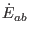# 6 Conclusion

The Weyl curvature tensor describes the nonlocal long-range interactions as enabling gravitational act at a distance (tidal forces and gravitational waves). The gravitoelectric field is described as the relativistic generalization of the tidal (Newtonian) force. However, the gravitomagnetic (anti-Newtonian) force has no Newtonian analogue. We have no expression similar toin the Newtonian theory. This difference arises from the instantaneous action in the Newtonian theory, which excludes a sounding solution. In Sec. 4, the rotation and distortion of the Weyl fields characterize the gravitational wave. The gravitomagnetism is necessary to maintain the gravitational wave. In relativistic models, the Newtonian force is also inconsistent without the magnetic part of the Weyl curvature.

Ashkbiz Danehkar
2018-03-26